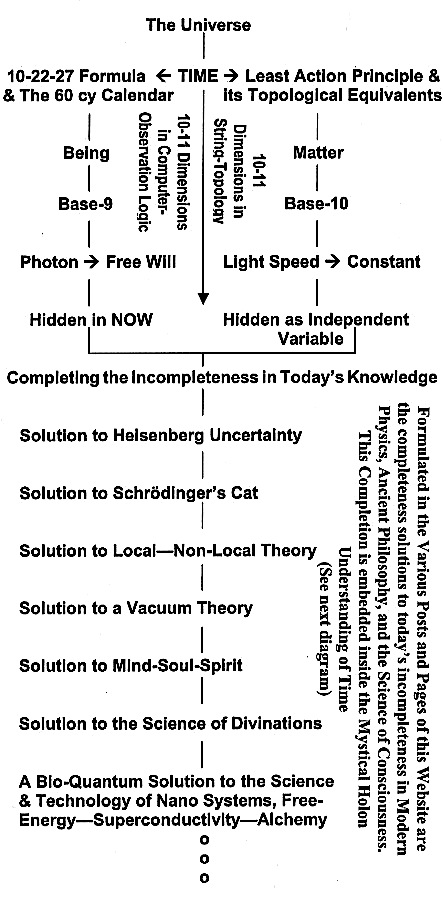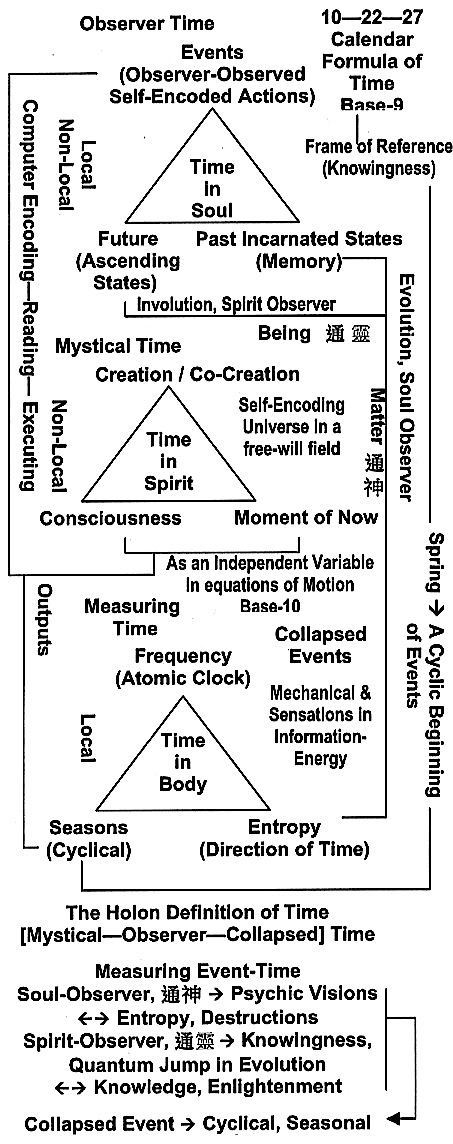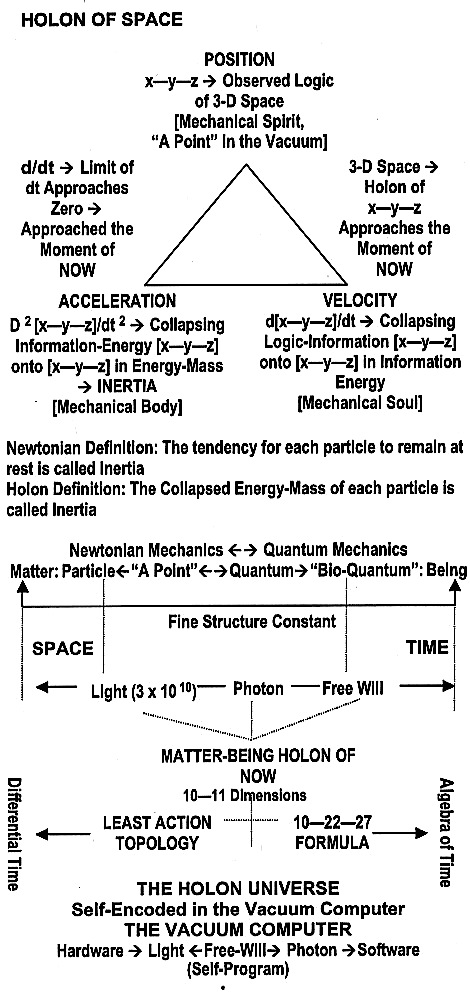* You are viewing the archive for the ‘Holon of Time-Space’ Category

## Holon of Time-Space

THE HOLON OF TIME

We are now almost at the end of my presentation on this Matter-Being Paradigm and this posting is an attempt to summarize or serve as a review of its essentials expressed within a Holon definition of Time. The Universe is formulated in the Mystical Holon definition of Time. It is the hidden Logic component of EVERYTHING. It Non-Locally Connects the Information of EVERYTHING with its particular Logic Component, and is the hidden Base 9 variable behind Bell’s Theorem, namely the variable that defines the Moment of NOW or Consciousness, which is also Co-Creation. Simultaneously, Time is also the hidden Base-10 variable in all equations of motion and thus forms a Non-Local—Local bridge between the Vacuum and the mechanical information of Space.

Remember, mechanical information is defined in this Paradigm as “anything or any variables (e.g., x, y, z)” that changes. Thus, the derivatives of these variables with time are the general formula for mechanical information. In this general case, Time is the Logic-Information component, while the x, y, z variables define the particle components. Their derivatives are the collapsed jointing of the two in the Moment of NOW. Thus, differential formulations are attempted to get to the Moment of NOW.

In conclusion, Time connects the Observer’s “Past” to the Observer’s “Future” with the Observer’s “Now” and thus establishes an Observer’s Participatory Universe. Remember, the “Past” and the “Future” include “Past Incarnations” and “Future Incarnations.” This is the logic embedded in the Science of Divination and my postulate of needing a shield for shielding “dis-information / entropic” free wills in the development of a deterministic Bio-Quantum and its Technology.

This understanding of Time is shown in the first diagram, while the Holon definition of Time is depicted in the second diagram below. Remember, all components within a Holon or Holons work together spirit—soul—mind/brain to form a unit of wholeness and this unit of wholeness is the Observer’s Reality constrained by the Observer’s Frame of Reference, which is a function of the Observer’s Knowledge and Knowingness.

Diagram 1

TIME, THE HIDDEN VARIABLEDiagram 2

THE HOLON OF TIMEPresented in this Website are two ways known by the author of formulating the local—Non-Local connection between the Spirit World and the Physical World. Both of these two ways are through the Base 9 and Base 10 formulas of Time.  The Base 9 formula is on the three Chinese Holons of Mind —–> --, Soul —–>--靈, and Spirit —–> 靈--. The interactions of these three Holons within the entire non-local—Local Base-9 Logic world are formulated in the Vacuum Computer by the 10—22—27 formula of time. Thus, their interactions must be formulated through computer Holon flow diagrams; rather than mathematical-topological equations.

On the other hand, the Base 10 formula of time is hidden as the independent variable of the equations, and its interactions are in mathematical-topologies (better yet, in Newtonian Mechanics). To understand either formula for time and its interactions, the proper frame of reference is needed, which implies the proper set of knowledge and knowingness.

In a geometrical metaphor, the Base 9 World is “inside” the circle, while the Base 10 World is outside the circle. Connecting these two worlds are variables Pi and Phi. As shown in previous postings, all the physical constants are generated by either or both Pi and Phi. Finally, one might conclude that the Soul World is the World formulated in Sacred Geometry. In short, the connections between these two formulas are sacred geometry, or the variables Pi and Phi, where all physical constants are generated (see previous postings). That is, Sacred Geometry connects the Base 9 World of the Spirit with the Base 10 World of the Body through the ever-changing World of the Soul in computer executions. Adding the collapsing logic that joints the two worlds, we have the Holon Pi—Phi—Li (Li —-> the Chinese Character ).

THE HOLON OF SPACE

Finally, with the above development on the mapping between Time and the Moment of NOW, we can now extend this understanding in the following computer flow-diagram formula for the Holon of Space. Obviously, both Time and Space are connected in the Moment of NOW via quantum collapses

Diagram 3Notice, embedded in light [or photons] are two connecting components, namely, electric [—–> Yang] and magnetic [—–> Yin]. These two components also contain the information of love [—–> positive-negative communications] and hate [—–> positive-positive / negative-negative communications].

The remaining of this posting will be concentrated on providing examples on practical applications of this 10—22—27 formula. First let us examine the 10—11 dimensional world with examples, whose interactions are formulated by this 10—22—27 formula.

[To Be Continue]